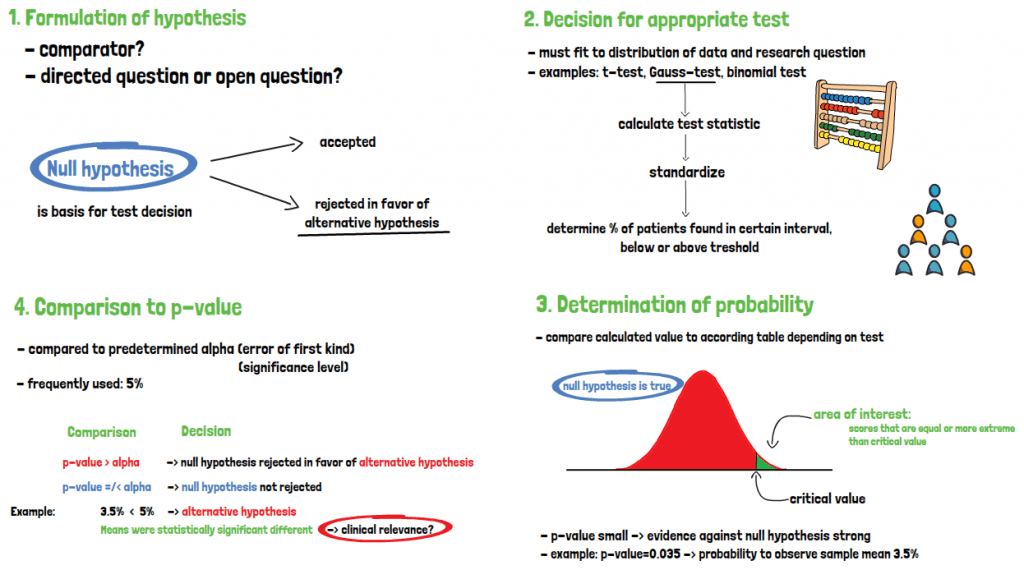# What is a significance test?A test of significance consists of four steps. First, the null and alternative hypothesis must be stated. Second, the test must be chosen appropriately, and the test statistic calculated. Third, the resulting p-value must be found in the test table. Finally, the p-value is compared to the alpha and a decision is made weather the null hypothesis is accepted or rejected in favor of the alternative hypothesis. Tests for statistical significance answer the question if an observed relationship might have occurred by chance.

The formulation of hypotheses depends on whether there is a comparator, as well as if the research question is asked in one direction only or if it is an open question addressing general change. A significance test is based on the null-hypothesis, which is either accepted or rejected in favor of the alternative hypothesis. No decision is made on whether the alternative hypothesis itself is true.

When the hypotheses are defined, the next step is to choose an appropriate statistical test that is fitting to the distribution of the data and the research question. With the chosen test, the test statistic can then be calculated. Standardization helps to determine the percentage of patients which are found in a certain interval or below or above a threshold. The probability of obtaining the value calculated and standardized under the assumption that the null hypothesis is true must be determined.

For that, the value must be compared to the according table depending on the test. As it is assumed that the null hypothesis is true, the area to the right of the critical value obtained from the table -when considering the normal distribution- is of interest. It is showing the probability of reaching scores that are equal or more extreme than the critical level obtained when the null-hypothesis is true. If the critical level is far away from the distribution mean, the p-value will also be small, which in return means that the evidence against the null-hypothesis is strong.

Finally, when the p-value is known, it must be compared to the predetermined alpha, that is the accepted error of the first kind, which is the error of falsely rejecting a null hypothesis, although it is in fact true. It is also called significance level. The frequently used threshold here is 5%. If the p-value is larger than alpha, the null hypothesis will not be rejected. If it is equal or smaller than alpha, the null hypothesis is rejected in favor of the alternative hypothesis. The importance of the result cannot be interpreted based on the p-value, only its statistical significance. But a statistically significant finding does not imply clinical relevance. Therefore, investigators should always consider both the statistical significance and the clinical relevance.

If you are interested in a visual presentation of this topic, please feel free to follow us on our GCP Mindset YouTube channel! If you would like to know more about how we could implement statistics in your clinical trial, send us a mail to statistics@gcp-service.com.